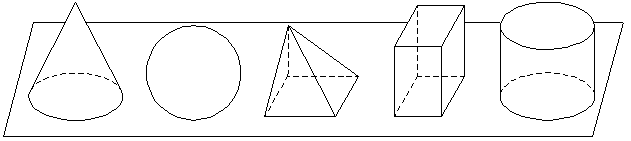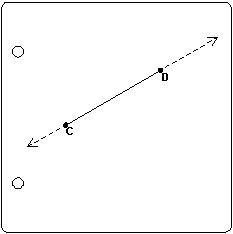This header plots the critical line of the Riemann Zeta Function.  A complete understanding wins a \$1,000,000 prize.
 . . .Main Links Orders Post Next Page Next + 10

CHAPTER 1
Planes, Points, and Lines

1.  You already know a great deal about geometry and geometric figures.  Since it may perhaps be difficult for you to recall these facts, we will help you by reviewing some of the material involving geometry which you have covered in your previous studies and which you have met in your everyday experiences.  First of all, we want you to test your ability to give to each of certain familiar objects the name of the geometric figure which describes the shape of that object.  To do this, we want you to use a simple matching test.

2.  Exercises:  Matching test.  In the two lists given below, match the geometric figures listed in Column II with the familiar objects listed in Column I.  For example, since a smooth-rimmed wheel is a circle, letter g is written in the parentheses next to number 1.  Also, since the flat surface of a desk is a plane, the letter i is written in the parentheses next to number 2.  Complete the test in a similar manner.

 I II 1. (g) A smooth-rimmed wheel a) Point 2. (i) The flat surface of a desk b) Line 3. (  ) The shape of the playing area of a football field c) Angle 4. (  ) The sharp end of a needle d) Right angle 5. (  ) The figure formed by the two hands of a clock at 3 o'clock e) Rectangle 6. (  ) The relation of an upright pole to the level ground f) Square 7. (  ) The four edges of the floor of a room which is just as long as it is wide g) Circle 8. (  ) A straight section of railroad track h) Perpendicular 9. (  ) The figure formed by the intersection of two spokes of a wheel i) Plane 10. (  ) The edge of a page of a book j) Parellel Lines 11. (  ) Part way around the rim of a cup k) Arc of a circle 12. (  ) A spoke of a wheel l) Radius of a circle

3. Answers to the above exercises.  To see how well you recalled the names of the geometric figures, compare your answers to the following: 1. g); 2. i); 3. e); 4. a); 5. d); 6. h); 7. f); 8. j); 9. c); 10. b); 11. k); 12. l).  Since these exercises covered only a few examples, each one of you may make up similar matching exercises using designs found on paper, on all kinds of cloth, on the walls of the room, in photographs of machinery, in advertisements, in building structures, in the designs from nature, and from other available sources.

4.  A plane.  Take a flat sheet of paper.  This flat sheet of paper represents a plane.  Of coursethe plane itself extends indefinitely beyond the four edges of the paper.  All the geometric figures with which you deal in Plane Geometry lie on a flat surface or plane.  The geometric figure which rest on a plane but which extend upward are figures used in Solid Geometry.  You are also acquainted with these figures because of your past experience.  To name only a few, you recall a cone, a sphere, a pyramid, a rectangular solid, and a cylinder.Cone, Sphere, Pyramid, Rectangular Solid, Cylinder
 5. Drawing and examining simple geometric figures:  A point and line. Again take a flat sheet of paper.  Put a dot any place on the paper and letter the dot A.  Put a second dot any other place on the paper and letter the dot B.  Let these dots represent points.  Take a ruler (or any convenient straightedge) and place it so that A and B lie along the straightedge and with a pencil draw the straight line AB.  We find that every time we draw a line from A to B along the straight edge of a ruler, the line lies exactly on AB.  So we say: Only one straight line can be drawn through two given points.6.  Postulates.  We call the above statement a postulate.  We did not prove the statement to be true, but every time we drew a straight line from A to B, we always got the same line.  So we assumed that only one straight line could be drawn from A to B.  We shall learn many postulates as the course develops, but here we will add only two more.  From the preceding figure we find:    1.  A straight line is the shortest distance between two points. And from the adjacent figure:    2.  A straight line can be extended indefinitely in either direction.7.  A line segment.  Although you know that a line can be extended, or produced, indefinitely, you deal frequently with only a portion of a line.  We call a portion of a straight line between two given points a segment or a line segment.  When the meaning in clear that we are dealing with a line segment, we often refer to it simply as a line.